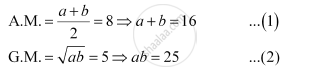CBSE (Arts) Class 11CBSE
Share

# If A.M. and G.M. of Roots of a Quadratic Equation Are 8 and 5, Respectively, Then Obtain the Quadratic Equation. - CBSE (Arts) Class 11 - Mathematics

ConceptRelationship Between A.M. and G.M.

#### Question

If A.M. and G.M. of roots of a quadratic equation are 8 and 5, respectively, then obtain the quadratic equation.

#### Solution

Let the root of the quadratic equation be a and b.

According to the given condition,The quadratic equation is given by,

x2– x (Sum of roots) + (Product of roots) = 0

x2 – x (a + b) + (ab) = 0

x2 – 16x + 25 = 0 [Using (1) and (2)]

Thus, the required quadratic equation is x2 – 16x + 25 = 0

Is there an error in this question or solution?

#### APPEARS IN

NCERT Solution for Mathematics Textbook for Class 11 (2018 to Current)
Chapter 9: Sequences and Series
Q: 32 | Page no. 193
Solution If A.M. and G.M. of Roots of a Quadratic Equation Are 8 and 5, Respectively, Then Obtain the Quadratic Equation. Concept: Relationship Between A.M. and G.M..
S